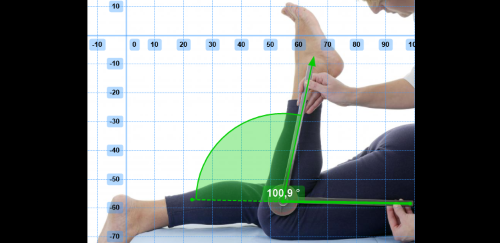Topic: Knee angles

Hey,

So I'm doing this sports science assignment and I got a bit confused. I'm measuring knee angles. The thing is that the deeper the person squats down, the smaller the angle is. As you can see in pic 1, the angle is 64.In pic 2 (look at the post below) you can see that the less you squat down, the bigger the angle is. In this case it's 93.

My question is: WHY DO I NEED TO SUBTRACT THE ANGLE FROM 180?

In pic 1 the answer would be 180-64= 116. The knee angle is equal 116.
In pic 2 180-93= 87

But why do I need to subtract? Can someone properly explain?

Sorry if this sounds like a stupid question but I'm confused

Re: Knee angles

Pic 2Re: Knee angles

Hi,
Please consider using the latest beta version from the download page, the angle tool is more versatile now and has a "supplementary angle" option that you can toggle in the menu which will give you the supplement to 180°.
You can also use the goniometer tool for a similar result to what you want.

The angles shown in the pictures are the actual angles formed by the two segments you put the angle on. Without more context the tool doesn't know anything else. Anatomical angles have various definitions depending on what is considered the anatomical rest position for the joint considered, in your case the 0° reference is when the leg is fully extended, so what you want to measure is the angle of the flexed leg relatively to the extended leg. This would not necessarily be the case if you wanted to measure the angle between the trunk and the thigh for example. It all depends on what is meant by 0° and in which direction the angle progresses.

See this image of the goniometer tool:The dashed line extending the horizontal leg of the angle would be your anatomical reference.